Download Presentation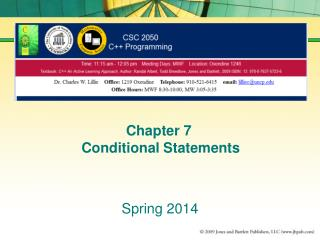Chapter 7 Conditional Statements

# Chapter 7 Conditional Statements - PowerPoint PPT Presentation

Chapter 7 Conditional Statements. Spring 2014. Chapter 7 Conditional Statements. 7.1 Conditional Expressions. Conditions - compare the values of variables, constants and literals using one or more relational operatorsI am the owner, or an agent authorized to act on behalf of the owner, of the copyrighted work described.
Download Presentation## Chapter 7 Conditional Statements

An Image/Link below is provided (as is) to download presentation

Download Policy: Content on the Website is provided to you AS IS for your information and personal use and may not be sold / licensed / shared on other websites without getting consent from its author.While downloading, if for some reason you are not able to download a presentation, the publisher may have deleted the file from their server.

- - - - - - - - - - - - - - - - - - - - - - - - - - E N D - - - - - - - - - - - - - - - - - - - - - - - - - -
Presentation Transcript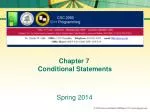### Chapter 7 Conditional Statements

Spring 2014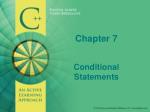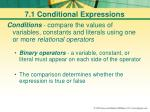7.1 Conditional Expressions
• Conditions - compare the values of variables, constants and literals using one or more relational operators
• Binary operators- a variable, constant, or literal must appear on each side of the operator
• The comparison determines whether the expression is true or false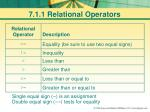7.1.1 Relational Operators
• Single equal sign (=) is an assignmentDouble equal sign (==) tests for equality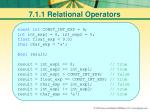7.1.1 Relational Operators
• constint CONST_INT_EXP = 9;
• int int_exp1 = 0, int_exp2 = 5;
• floatfloat_exp = 9.0;
• char char_exp = 'a';
• bool result;
• result = int_exp1 == 0;// true
• result = int_exp2 >= int_exp1;// true
• result = int_exp1 > CONST_INT_EXP;// false
• result = float_exp == CONST_INT_EXP;// true
• result = char_exp <= int_exp1;// false result = int_exp1 != int_exp2;// true
• result = char_exp == 'a';// true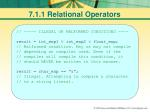7.1.1 Relational Operators
• // ----- ILLEGAL OR MALFORMED CONDITIONS ----
• result = int_exp1 < int_exp2 < float_exp;
• // Malformed condition. May or may notcompile // depending on compiler used. Even if the// compiler will compile this condition, it // should NEVER be written in this manner.
• result = char_exp == "a";
• // Illegal. Attempting to compare a character// to a string literal.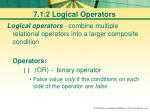7.1.2 Logical Operators
• Logical operators - combine multiple relational operators into a larger composite condition
• Operators:

|| (OR) - binary operator

• False value only if the conditions on each side of the operator are false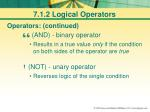7.1.2 Logical Operators
• Operators: (continued)

&& (AND) - binary operator

• Results in a true value only if the condition on both sides of the operator are true

! (NOT) - unary operator

• Reverses logic of the single condition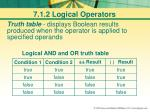7.1.2 Logical Operators
• Truth table-displays Boolean results produced when the operator is applied to specified operands
• Logical AND and OR truth table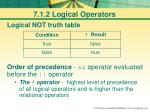7.1.2 Logical Operators
• Logical NOT truth table
• Order of precedence - && operator evaluated before the || operator
• The ! operator - highest level of precedence of all logical operators and is higher than the relational operators7.1.2 Logical Operators
• Misc Information:
• Parentheses change the precedence
• Parentheses can help clarify complicated conditions
• Short-circuit evaluation - once the outcome of condition can be determined, evaluation ends7.1.2 Logical Operators
• Various logical operators
• int int_exp1 = 0, int_exp2 = 5;
• float float_exp = 9.0;
• charchar_exp = 'a';
• constintCONST_INT_EXP = 9;
• bool result;
• result = int_exp1 < int_exp2 && float_exp == 9.0; // true
• result = int_exp1 > CONST_INT_EXP || float_exp == 9.0;// true
• result = !(float_exp == 9.0 || int_exp1 > CONST_INT_EXP);// false
• // Short-Circuit Evaluation
• result = float_exp == 9.0 || int_exp1 > CONST_INT_EXP; // true

T

T

T

T

F

T

T

F

F

T

T

T

Not Evaluated7.2 The if Statement
• if statement - uses conditions to determine a specific action
• Syntax:if ( <condition> )
• <action>7.2 The if Statement
• <condition> -any valid expression, either built from relational and logical operators or from evaluation of a single variable
• zero is false while any non-zero value is considered true

<action> -any valid C++ statement

• multiple statements must be enclosed in curly braces { }7.2 The if Statement
• if statement - uses conditions to determine a specific action
• // Example 1
• if ( test >= 80 && test < 90 )
• cout << "You have earned a B" << endl;// Action block
• // Example 2
• if( test >= 90 )
• {// Start of the action block
• cout << "You have earned an A" << endl;
• cout << "Excellent work!" << endl;
• }// End of the action block
• // Example 3
• if( test >= 70 && test < 80 )
• {// Start of the action block
• cout << "You have earned a C" << endl;
• }// End of the action block7.2.1 The else Statement
• else statement - optional part of if statement
• Can’t stand alone
• Must be associated with an if
• if( <condition> )
• <action 1>
• else
• <action 2>7.2.1 The else Statement
• else
• no condition or expression associated with it
• relies on results of the condition associated with the if
• executes action(s) only if the condition is false
• action can contain one or more statements
• if more than one statement, the action must be enclosed in curly braces7.2.1 The else Statement
• else Example
• if ( grade >= 60 )
• pass = true;
• else
• {
• pass =false;
• cout << "Hope you do better next time" << endl;
• }7.2.1 The Nested if
• Nested if - embedding another ifin action block of the else
• if( avg >= 90 )
• cout << "A" << endl;
• else
• if ( avg >= 80 )
• cout << "B" << endl;7.2.1 The Nested if
• Nested if indentation can cause the code to become difficult to read
• if ( <condition 1> )
• <action 1>
• else if ( <condition 2> )
• <action 2>
• else if ( <condition 3> )
• <action 3>
• . . .
• else // Optional
• <last action>7.2.1 The Nested if
• Inefficient if statement
• if ( avg >= 90 )
• cout << "A" << endl;
• if ( avg >= 80 && avg < 90 )
• cout << "B" << endl;
• if ( avg >= 70 && avg < 80 )
• cout << "C" << endl;
• if ( avg >= 60 && avg < 70 )
• cout <<"D" << endl;
• if ( avg < 60 )
• cout << "F" << endl;7.2.1 The Nested if
• Using else if statements is much more efficient than using separate if statements
• else if statement
• if ( avg >= 90 )
• cout << "A" << endl;
• elseif ( avg >= 80 )
• cout << "B" << endl;
• elseif( avg >= 70 )
• cout << "C" << endl;
• elseif ( avg >= 60 )
• cout << "D" << endl;
• else
• cout << "F" << endl;7.2.1 The Nested if
• Why is else if more efficient?
• else if only evaluates if condition until it finds true
• Inefficient if evaluates every if condition
• if ( avg >= 90 )
• cout << "A" << endl;
• if ( avg >= 80 && avg < 90 )
• cout << "B" << endl;
• if ( avg >= 70 && avg < 80 )
• cout << "C" << endl;
• if ( avg >= 60 && avg < 70 )
• cout <<"D" << endl;
• if ( avg < 60 )
• cout << "F" << endl;
• if ( avg >= 90 )
• cout << "A" << endl;
• elseif ( avg >= 80 )
• cout << "B" << endl;
• elseif( avg >= 70 )
• cout << "C" << endl;
• elseif ( avg >= 60 )
• cout << "D" << endl;
• else
• cout << "F" << endl;7.2.1 The Nested if
• Flow of an if statement7.2.1 The Nested if
• Nested control statement - has another control statement in its action block
• Map most nested if statement with nearest unmatched else
• if ( gpa >= 3.75 )
• if ( credits > 25 )
• if( money < 30000 )
• { scholarship = 5000;
• cout <<"Way to go!" << endl;
• }
• else
• scholarship = 2000;
• else
• scholarship = 1000;
• else
• { scholarship = 0;
• cout << "You're on your own."<< endl;
• }7.3 Variable Scope
• Scope of a variable – determines:
• What code can access or change the variable
• How long the variable exists or lives7.3 Variable Scope
• Below, var_a and var_b defined within the scope of the block
• Both accessible within the block where defined
• Final line generates an error message - var_b is not defined
• {
• intvar_a = 5, var_b = 10;
• var_a++;
• cout <<"var_a: "<< var_a << endl;
• }
• cout <<"var_b: "<< var_b;// Error: undeclared // identifiervar_b7.3 Variable Scope
• Local scope – variables or constants declared within braces7.3 Variable Scope
• Constant PI and variable global_area - physically declared outside of function - placed at the global level
• #include<iostream>
• using std::cout;
• using std::endl;
• #include<cmath>// Needed for pow
• constfloat PI = 3.141592F;// global scope
• floatglobal_area = 0; // global scope
• intmain()
• {floatradius = 5;// local scope
• global_area =static_cast<float>( PI* pow( radius, 2 ) );
• cout << global_area <<" sq. in."<< endl;
• return0;
• }
• // Output
• 78.5398 sq. in.7.3 Variable Scope
• Any code within the file can access PI or global_area
• #include<iostream>
• using std::cout;
• using std::endl;
• #include<cmath>// Needed for pow
• constfloat PI = 3.141592F;// global scope
• floatglobal_area = 0; // global scope
• intmain()
• {floatradius = 5; // local scope
• global_area =static_cast<float>(PI* pow(radius, 2));
• cout << global_area <<" sq. in."<< endl;
• return0;
• }
• // Output
• 78.5398 sq. in.7.3 Variable Scope
• Global variables - automatically initialized to 0

Avoid global variables (i.e., global_area)

• #include<iostream>
• using std::cout;
• using std::endl;
• #include<cmath>// Needed for pow
• constfloat PI = 3.141592F;// global scope
• floatglobal_area = 0; // global scope
• intmain()
• {floatradius = 5; // local scope
• global_area =static_cast<float>(PI* pow(radius, 2));
• cout << global_area <<" sq. in."<< endl;
• return0;
• }
• // Output
• 78.5398 sq. in.7.4 The switch Statement
• switch statement - another form of conditional statement
• Also called a selection statement
• Checks only for equality and only for one variable7.4 The switch Statement
• Works well for checking a variable for limited set of values
• Only works with ordinal data types
• Ordinal data types - can be translated into an integer to provide a finite, known, number set
• Examples include int, bool, char, andlong7.4 The switch Statement
• General form of the switch statement:
• switch( <variable> )
• { // Required
• case <literal or const 1>:
• <action 1>
• break;
• case <literal or const 2>:
• <action 2>
• break;
• ...
• default: // Optional
• <default action>
• }// Required
• When first line is encountered, value of the variable determined
• Execution jumps to the case which corresponds to the value of the variable being examined
• Execution continues until either a break statement is encountered or to the end of switch7.4 The switch Statement
• break statement - stops execution of the control structure prematurely
• Stops multiple case statements from being executed
• Many believe poor programming to use outside the context of the switch statement7.4 The switch Statement
• default statement - executed if value of the variable doesn’t match any of previous cases
• Type of catch all or “case else”
• Technically can use the default case in any position
• Should physically be the last one in the switch statement7.4 The switch Statement
• intmenu_item = 0;
• ...
• switch ( menu_item )
• {
• case1: // Using literal values
• cout << "You have chosen option 1." << endl;
• break;
• case2:
• cout << "You have chosen option 2." << endl;
• break;
• case3:
• cout << "You have chosen option 3." << endl;
• break;
• default:
• cout << "Invalid menu option." << endl;
• }7.4 The switch Statement
• constshortGREEN = 0;
• constshortYELLOW = 1;
• constshortRED = 2;
• shortlight_color = GREEN;
• switch ( light_color )
• { caseGREEN: // Using constants
• cout <<"Go!"<< endl;
• break;
• caseYELLOW:// Let fall through
• caseRED:
• cout <<"Stop!";
• cout <<"Proceed when light is green."<< endl;
• break;
• default:
• cout <<"Stop!";
• cout <<"Power is out!"<< endl;
• }7.4 The switch Statement
• cout <<"Enter letter grade: ";
• cin >> letter_grade;
• switch ( letter_grade )
• { case'A': // Using character literal values
• cout <<"Excellent!"<< endl;
• break;
• case'B':
• cout <<"Above average." << endl;
• break;
• case'C':
• cout <<"Average."<< endl;
• break;
• case'D':
• cout <<"Below average."<< endl;
• break;
• case'F':
• cout <<"Failed!"<< endl;
• break;
• default:
• cout <<"Invalid letter grade."<< endl;
• }7.4 The switch Statement
• One of the most common uses of switch statement is in menu driven programs
• Student Grade Program - Main Menu -
• 1. Enter name 2. Enter test scores
• 3. Display test scores
• 9. Exit
• Please enter your choice from the list above:7.5 Conditional Operator
• Conditional operator- considered a ternary operator, meaning it has three operands
• Syntax:

<condition> ? <true expression> : <false expression>7.5 Conditional Operator
• One of the expressions is returned based upon the evaluation of the condition
• int a = 5, b = 0;
• intlarger = a > b ? a : b;
• cout << larger << endl;
• // Output
• 57.5 Conditional Operator
• Equivalent if statement to code on previous page
• int a = 5, b = 0;
• intlarger;
• if ( a > b )
• larger = a;
• else
• larger = b;7.5 Conditional Operator
• More challenging conditional operator example
• short hour = 9, minute = 10, second = 5;
• cout << (hour < 10 ?"0" :"") << hour <<":"
• << (minute < 10 ?"0":"")<< minute <<":"
• << (second < 10 ?"0":"")<< second << endl;
• // Output
• 09:10:05
• Empty quotes above tell cout to print nothing if the condition is false (i.e. hour is 10 or greater)7.7 C – The Differences
• Previous versions of C did not have a Boolean data type
• There isn’t a predefined true or false
• All relational operators return either a zero for false or a non-zero value, usually one, for true7.7 C – The Differences
• C programmers often create their own Boolean data type as shown below
• The C99 version of the ANSI Standard includes a Boolean data type
• Not currently supported by Visual Studio
• #defineBOOLint
• #defineTRUE 1
• #defineFALSE 0
• intmain(void)
• { BOOL done = FALSE;
• return0;
• }